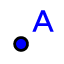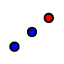# 29 September 2020

## A new version of GeoGebra Discovery with improved symbolic angle bisectors

After several years of doubt and confusion I finally managed to find a way to speed up symbolic computations related to angle bisectors and incircles. There are several approaches to decribe angular bisectors symbolically but many of them can lead to heavy computations.

For this reason GeoGebra has no effective way to prove anything difficult related to angle bisectors and incircles in a triangle in the plane. After several discussions with my former advisor Tomás Recio I learned that a certain approach will actually help. I re-read his 2009 paper (co-authored with Dalzotto) and found that not just for angle bisectors but also for the center of an incircle there are already well-working methods.

I leave the details to the expert readers (see pages 231 and 226 for the tricks in the Dalzotto-Recio paper). Here I only illustrate the speedup from the user's perspective—so I will be as practical as possible.

### Testing if a command gives the correct symbolic output

Two tests are required. In both tests we use the geometric command and try to force using it symbolically. First of all, we create a triangle and use the newly implemented Incircle tool to create its incircle (select the iconin the 6. toolset). You need to click on the vertices of the triangle to get the incircle.

Now put a point on the circle by selecting the Point tool (from the second toolset) and reflect the attached point about a vertex of the triangle (say, $$A$$). (To do that, use the Reflect about Point toolfrom the 9th toolset.) Finally, to learn if the symbolic result is correct, type the command LocusEquation(D',D), this should work if $$D'$$ is the reflected point and $$D$$ lies on the circle. The expected output is a circle, because in this case GeoGebra simply takes the numerical equation of the circle (computed by the Incircle tool numerically) and converts back to symbolic equation, and finally the geometric object defined by this equation will be “reflected”.

You may want to change the color of the symbolic locus object. This can be done easily by clicking on the icon on the top-right corner of the Graphics View, and after selecting the locus object, a different color can be chosen.

But we need a second test to check if the symbolic representation of the incircle is correct.

When point $$P$$ is running on path $$\ell$$, a perpendicular line $$p$$ is sweeping the plane. That is, intersection points $$I$$ and $$J$$ will cover the complete set of the locus. In this case GeoGebra no longer uses the numerical form of the input path, but its symbolic form will be used. Therefore, the command LocusEquation(I,P) (or, LocusEquation(J,P), it has the same meaning) will show the actual symbolic locus.

Note that not only the incircle is shown, but also 3 excircles. In fact, there is no way to use complex algebraic geometry to distinguish between these 4 circles. So all of them are handled at the same time without making any difference between them.

### Visualizing Euler's Inequality

It is well-known that in all planar triangles an inequality holds, namely $$R\ge2r$$ where $$R$$ denotes the circumradius and $$r$$ stands for the radius of the incircle.

The new IncircleCenter tool and command helps visualizing this faster than before.

(If you cannot see the red locus, please drag the points $$A$$ or $$B$$ a bit.)

The red points show where the equality $$R=2r$$ holds if point $$C$$ is placed on the curve (it is an octic, that is, of degree 8). In fact, $$r$$ should be displayed 4 times in this picture, because the equality holds only in one case for the incircle (namely, if the triangle is equilateral), but infinitely many times if $$r$$ represents the radius of an excircle. In a future version of this applet the excircles should also be drawn—now your fantasy is required to make it complete.

You can test your machine's speed by dragging $$A$$ or $$B$$. Expect slow motion, even on fast machines. For further reading the following literature is recommended:

### Just bisectors and nothing more: Steiner-Lehmus variations

With the fast bisector computation algorithm the road has been opened to visualize variations of the Steiner-Lehmus theorem in a browser. In complex algebraic geometry, as mentioned above, internal and external angle bisectors cannot be distinguished—so we need to consider all combinations of them when studying the length of the angle bisectors.

In this final applet we ask GeoGebra Discovery to find all of those triangles where the ratio of the lengths of the angle bisectors is $$1:2$$. So by entering the command LocusEquation(AD==2BE,C) we get the following output:

(If you cannot see the red locus, please drag the points $$A$$ or $$B$$ a bit.)

Again, this is a heavy applet. Your machine needs to be powerful enough to get an enjoyable motion if you drag points $$A$$ or $$B$$.

In fact, this curve consists of two components. A single component is the red line that connects $$A$$ and $$B$$. The other component is $\displaylines{144 \; x^{14} + 1920 \; x^{13} + 783 \; x^{12} \; y^{2} - 62720 \; x^{12} + 7440 \; x^{11} \; y^{2} - 184320 \; x^{11} + 1674 \; x^{10} \; y^{4} \\ - 269600 \; x^{10} \; y^{2} + 6361088 \; x^{10} + 8400 \; x^{9} \; y^{4} + 165120 \; x^{9} \; y^{2} - 491520 \; x^{9} + 1665 \; x^{8} \; y^{6} \\ - 417504 \; x^{8} \; y^{4} + 12022016 \; x^{8} \; y^{2} - 253034496 \; x^{8} - 2400 \; x^{7} \; y^{6} + 1981440 \; x^{7} \; y^{4} - 61071360 \; x^{7} \; y^{2} \\ + 346030080 \; x^{7} + 540 \; x^{6} \; y^{8} - 228416 \; x^{6} \; y^{6} - 5737472 \; x^{6} \; y^{4} + 411320320 \; x^{6} \; y^{2} + 4717543424 \; x^{6} - 12000 \; x^{5} \; y^{8} \\ + 3110400 \; x^{5} \; y^{6} - 81469440 \; x^{5} \; y^{4} - 772669440 \; x^{5} \; y^{2} - 10192158720 \; x^{5} - 351 \; x^{4} \; y^{10} + 64576 \; x^{4} \; y^{8} - 24287744 \; x^{4} \; y^{6} \\ + 1287208960 \; x^{4} \; y^{4} - 19060948992 \; x^{4} \; y^{2} - 39845888000 \; x^{4} - 8880 \; x^{3} \; y^{10} + 1858560 \; x^{3} \; y^{8} + 1597440 \; x^{3} \; y^{6} - 5343805440 \; x^{3} \; y^{4} \\ + 134936002560 \; x^{3} \; y^{2} + 116769423360 \; x^{3} - 342 \; x^{2} \; y^{12} + 116064 \; x^{2} \; y^{10} - 15080448 \; x^{2} \; y^{8} + 555204608 \; x^{2} \; y^{6} + 9068216320 \; x^{2} \; y^{4} \\ - 371399327744 \; x^{2} \; y^{2} + 90999619584 \; x^{2} - 2160 \; x \; y^{12} + 380160 \; x \; y^{10} + 22487040 \; x \; y^{8} - 1850081280 \; x \; y^{6} - 2972712960 \; x \; y^{4} + 460786237440 \; x \; y^{2} \\ - 483183820800 \; x - 81 \; y^{14} + 33696 \; y^{12} - 2191104 \; y^{10} + 8110080 \; y^{8} + 1524695040 \; y^{6} - 3529506816 \; y^{4} - 211543916544 \; y^{2} + 347892350976,}$ an irreducible polynomial of degree 14 (it is irreducible over $$\mathbb{R}$$).

See also a nice CindyJS/WebGL applet for a faster numerical exploration (for the original experiment where the ratio of the lengths is $$1:1$$).

### What next?Zoltán Kovács Linzer Zentrum für Mathematik Didaktik Johannes Kepler Universität Altenberger Strasse 54 A-4040 Linz